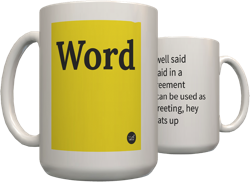Top definition
The representation of a zero followed by an infinite number of decimal three's. Can also be written as "0.333.." This number is exactly equal to 1/3, a fact that people with weak mathematical understanding usually don't get.

This "controversy" has a tendency to appear again and again in various forums and discussions and places on the net, to the annoyance of everyone.

Proof:

x = 0.333..
10x = 3.333..
10x - x = 3.333.. - 0.333..
9x = 3
x = 1/3
Noooo, not the 0.333... debate AGAIN!
by Skrolle July 01, 2005Get a .3~ mug for your Aunt Larisa.
2
.3~ or .3 repeating is commonly thought to equal 1/3. Believers of this idea claim that because the number of decimals is infinite, that it must equal 1/3. They sometimes use the supporting example that .33333333=1/3, therefore .66666666=2/3 therefore .99999999= 3/3, or 1. To prove this wrong, you need to consider 2 things. First, and most sensible, 10 is not divisible by 3. Therefore, no matter how many .3's you use, they will never be able to complete the whole number 1. The second thing you need to consider is that with .33333~, you will always be off by just a little from achieving 1/3. This is why when you use a calculator and enter 1/3, the decimal given is .333333334.
.33333333x3= .99999999
.33333334x3=1.02
The answer of what decimal multiplied by 3 equals one is lurking somewhere in between those 2.
by Don June 20, 2005